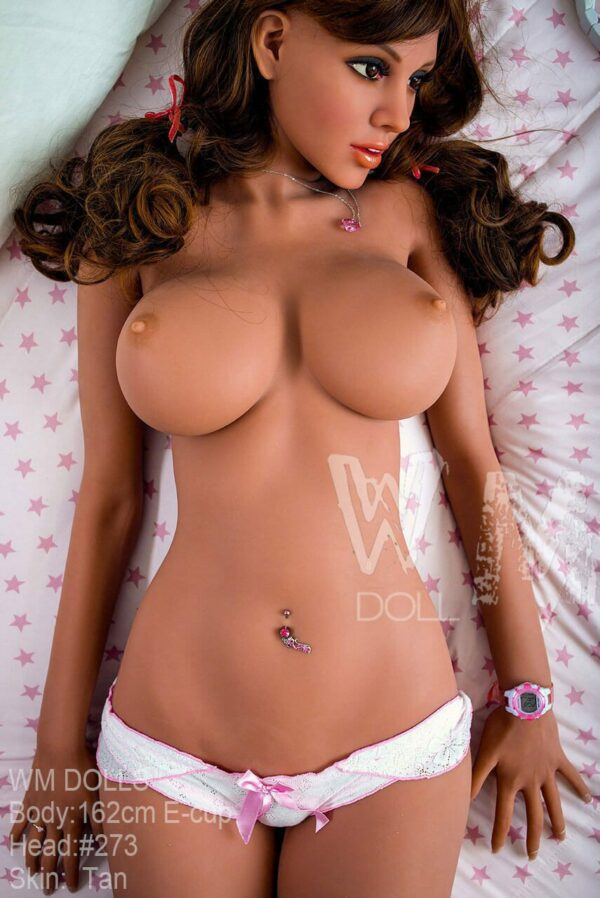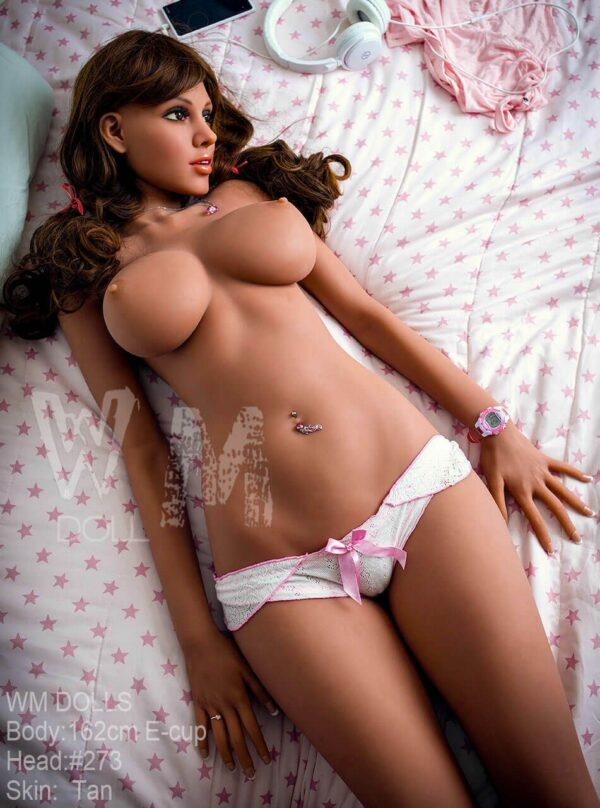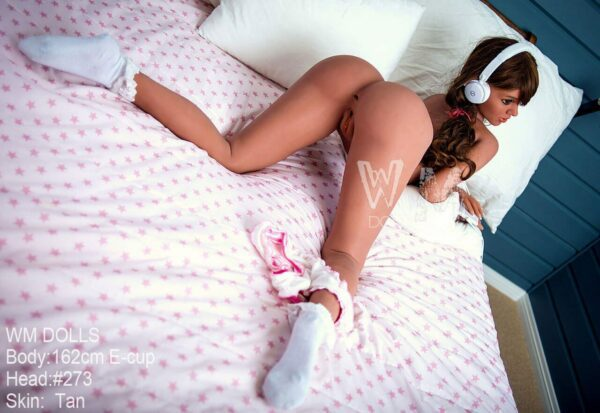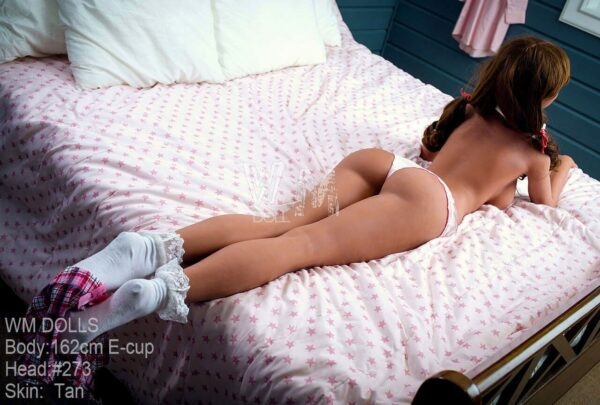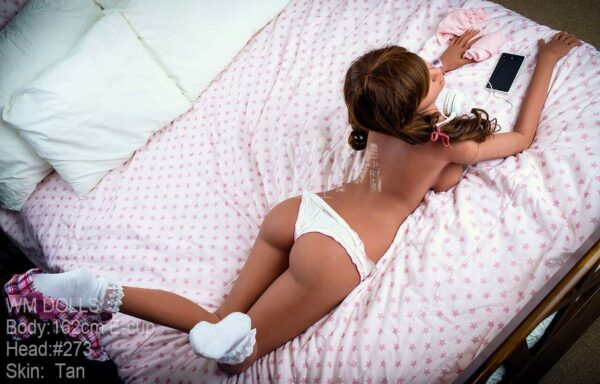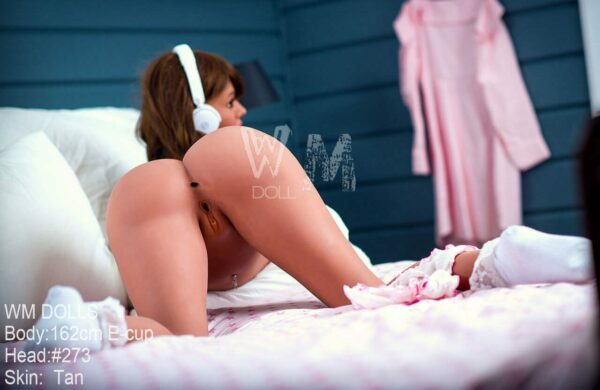# Celia – 5’4″ | 162cm WM Sex Doll

WM Doll
\$1,700

Celia – 5’4″ | 162cm WM Sex Doll made with high quality and safety standards by WM Doll, this genuine silicone and TPE sexy real sex doll is CE certified. With various features complementing each other, this realistic sex doll is sure to please the owner.

## Description

Height > 5ft 3.5 in – 5ft 5in (161cm – 165cm), Ethnicity > American, Body Features > Big Lips, Hair Color > Brunette, Material > Fully TPE, Breast Size > Large, Ethnicity > Latina, Luxury Sex Dolls, Breast Size > Medium, See All Sex Dolls, Body Style > Slender, BRANDS > WM Doll, Age > Young Adult

• \$
• \$
• \$
• \$
• \$
• \$
• \$
• \$
• \$
• \$
• \$
• \$
• \$
• \$
• \$
• \$
• \$
• \$
• \$
• \$
• \$
• \$
• \$
• \$
• \$
• \$
• \$
• \$
• \$
• \$
• \$
• \$
• \$
• \$
• \$
• \$
• \$
• \$
• \$
• \$
• \$
• \$
• \$
• \$
• \$
• \$
• \$
• \$
• \$
• \$
• \$
• \$
• \$
• \$
• \$
• \$
• \$
• \$
• \$
• \$
• \$
• \$
• \$
• \$
• \$
• \$
• \$
• \$
• \$
• \$
• \$
• \$
• \$
• \$
• \$
• \$
• \$
• \$
• \$
• \$
• \$
• \$
• \$
• \$
• \$
• \$
• \$
• \$
• \$
• \$
• \$
• \$
• \$
• \$
• \$
• \$
• \$
• \$
• \$
• \$
• \$
• \$
• \$
• \$
• \$
• \$
• \$
• \$
• \$
• \$
• \$
• \$
• \$
• \$
• \$
• \$
• \$
• \$
• \$
• \$
• \$
• \$
• \$
• \$
• \$
• \$
• \$
• \$
• \$
• \$
• \$
• \$
• \$
• \$
• \$
• \$
• \$

• \$
• \$
• \$
• \$
• \$
• \$
• \$

• \$

• \$
• \$
• \$
• \$
• \$
• \$
• \$
• \$
• \$
• \$
• \$
• \$
• \$
• \$
• \$
• \$
• \$
• \$
• \$
• \$
• \$

• \$
• \$
• \$
• \$
• \$
• \$
• \$
• \$
• \$
• \$
• \$
• \$
• \$
• \$
• \$
• \$
• \$
• \$
• \$
• \$

• \$
• \$
• \$
• \$
• \$
• \$
• \$

• \$
• 40 \$

• \$
• 100 \$

• \$
• \$
• \$
• \$
• \$

• \$
• \$
• \$
• \$
• \$

• \$
• \$
• \$

• \$
• 130 \$

• \$
• \$
• \$

• \$
• 40 \$

• \$
• \$
• \$
• \$

• \$
• \$
• \$
• \$
• \$

• \$
• \$

• \$
• \$
• \$
• \$
• \$
• \$
• \$
• \$
• \$
• \$

• \$
• \$
• \$
• \$
• \$
• \$

• \$
• 200 \$

• \$
• 100 \$

• \$
• 675 \$

• \$
• 390 \$

## Doll Measurements

Height: 162 cm | 5 ft 3.7 in
Weight: 35kg | 77.1 lbs.
Full Bust: 81 cm | 31.8 in
Cup Size: E
Waist: 57 cm | 22.4 in
Hips: 91 cm | 35.8 in
Foot Length: 22 cm | 8.2 in
Vaginal Depth: 18cm | 7 in
Anal Depth: 15 cm | 5.9 in
Oral Depth: 12 cm | 4.7 cm